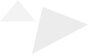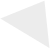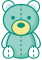# Python实战|利用Dowhy框架实现因果推断实战（二）1.Dowhy因果推断框架

2.数据来源及预处理

3.数据相关性探索

1.计算期望频数，初步判断因果关系

2.基于假设创建因果图

3.识别因果效应

4.估计因果效应

5.反驳结果# 因果推断实现

## 1.计算期望频数，初步判断因果关系

counts_sum=0
for i in range(1,10000):
counts_i = 0
rdf = data.sample(1000)
counts_i =rdf[rdf["is_canceled"]==rdf["different_room_assigned"]].shape
counts_sum+= counts_i
counts_sum/10000

517.9752


counts_sum=0
for i in range(1,10000):
counts_i = 0
rdf =data[data["booking_changes"]==0].sample(1000)
counts_i =rdf[rdf["is_canceled"]==rdf["different_room_assigned"]].shape
counts_sum+= counts_i
counts_sum/10000

492.0499


counts_sum=0
for i in range(1,10000):
counts_i = 0
rdf =data[data["booking_changes"]>0].sample(1000)
counts_i =rdf[rdf["is_canceled"]==rdf["different_room_assigned"]].shape
counts_sum+= counts_i
counts_sum/10000
663.4134